Skip to main content Accessibility help
Home
Hostname: page-component-684bc48f8b-4z9h4 Total loading time: 1.274 Render date: 2021-04-11T12:21:16.799Z Has data issue: true Feature Flags: { "shouldUseShareProductTool": true, "shouldUseHypothesis": true, "isUnsiloEnabled": true, "metricsAbstractViews": false, "figures": false, "newCiteModal": false, "newCitedByModal": true }Compositio Mathematica

# Sup-norms of eigenfunctions in the level aspect for compact arithmetic surfaces, II: newforms and subconvexity

Published online by Cambridge University Press:  15 December 2020

Corresponding
E-mail address:

## Abstract

We improve upon the local bound in the depth aspect for sup-norms of newforms on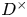$D^\times$, where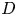$D$ is an indefinite quaternion division algebra over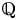${\mathbb {Q}}$. Our sup-norm bound implies a depth-aspect subconvexity bound for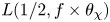$L(1/2, f \times \theta _\chi )$, where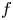$f$ is a (varying) newform on$D^\times$ of level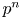$p^n$, and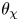$\theta _\chi$ is an (essentially fixed) automorphic form on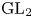$\textrm {GL}_2$ obtained as the theta lift of a Hecke character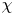$\chi$ on a quadratic field. For the proof, we augment the amplification method with a novel filtration argument and a recent counting result proved by the second-named author to reduce to showing strong quantitative decay of matrix coefficients of local newvectors along compact subsets, which we establish via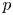$p$-adic stationary phase analysis. Furthermore, we prove a general upper bound in the level aspect for sup-norms of automorphic forms belonging to any family whose associated matrix coefficients have such a decay property.

## MSC classification

Type
Research Article
Information
Compositio Mathematica , November 2020 , pp. 2368 - 2398
Copyright
© The Author(s) 2020

## Access options

Get access to the full version of this content by using one of the access options below.

## References

Assing, E., On the size of$p$-adic Whittaker functions, Trans. Amer. Math. Soc. 372 (2019), 52875340.CrossRefGoogle Scholar
Blomer, V., Epstein zeta-functions, subconvexity, and the purity conjecture, J. Inst. Math. Jussieu 19 (2018), 116.Google Scholar
Blomer, V., Harcos, G., Maga, P. and Milićević, D., The sup-norm problem for$\textrm {GL}(2)$ over number fields, J. Eur. Math. Soc. (JEMS) 22 (2020), 153.CrossRefGoogle Scholar
Blomer, V. and Holowinsky, R., Bounding sup-norms of cusp forms of large level, Invent. Math. 179 (2010), 645681.CrossRefGoogle Scholar
Bushnell, C. J. and Henniart, G., The local Langlands conjecture for GL(2) (Springer, Berlin, 2006).CrossRefGoogle Scholar
Corbett, A. and Saha, A., On the order of vanishing of newforms at cusps, Math. Res. Lett. 25 (2018), 17711804.CrossRefGoogle Scholar
File, D., Martin, K. and Pitale, A., Test vectors and central$L$-values for$\textrm {GL}(2)$, Algebra Number Theory 11 (2017), 253318.CrossRefGoogle Scholar
Gelbart, S., Automorphic forms on adèle groups, Annals of Mathematics Studies, vol. 83 (Princeton University Press–University of Tokyo Press, Princeton, NJ–Tokyo, 1975).CrossRefGoogle Scholar
Gross, B. H., Local orders, root numbers, and modular curves, Amer. J. Math. 110 (1988), 11531182.CrossRefGoogle Scholar
Harcos, G. and Michel, P., The subconvexity problem for Rankin–Selberg$L$-functions and equidistribution of Heegner points. II, Invent. Math. 163 (2006), 581655.CrossRefGoogle Scholar
Harcos, G. and Templier, N., On the sup-norm of Maass cusp forms of large level. II, Int. Math. Res. Not. IMRN 2012 (2012), 47644774.CrossRefGoogle Scholar
Harcos, G. and Templier, N., On the sup-norm of Maass cusp forms of large level. III, Math. Ann. 356 (2013), 209216.CrossRefGoogle Scholar
Harris, M. and Kudla, S. S., The central critical value of a triple product$L$-function, Ann. of Math. (2) 133 (1991), 605672.CrossRefGoogle Scholar
Hu, Y., Triple product formula and the subconvexity bound of triple product$L$-function in level aspect, Amer. J. Math. 139 (2017), 215259.CrossRefGoogle Scholar
Hu, Y., Triple product formula and mass equidistribution on modular curves of level$N$, Int. Math. Res. Not. IMRN 2018 (2018), 28992943.Google Scholar
Hu, Y. and Nelson, P. D., New test vector for Waldspurger's period integral, Preprint (2018), arXiv:1810.11564v1.Google Scholar
Hu, Y., Nelson, P. D. and Saha, A., Some analytic aspects of automorphic forms on$\textrm {GL}(2)$ of minimal type, Comment. Math. Helv. 94 (2019), 767801.CrossRefGoogle Scholar
Humphries, P. and Khan, R., On the random wave conjecture for dihedral Maaß forms, Geom. Funct. Anal. 30 (2020), 34125.CrossRefGoogle Scholar
Iwaniec, H. and Kowalski, E., Analytic number theory, American Mathematical Society Colloquium Publications, vol. 53 (American Mathematical Society, Providence, RI, 2004).Google Scholar
Iwaniec, H. and Sarnak, P.,$L^{\infty }$ norms of eigenfunctions of arithmetic surfaces, Ann. of Math. (2) 141 (1995), 301320.CrossRefGoogle Scholar
Kowalski, E., Michel, P. and VanderKam, J., Rankin–Selberg$L$-functions in the level aspect, Duke Math. J. 114 (2002), 123191.CrossRefGoogle Scholar
Marshall, S., Local bounds for$L^p$ norms of Maass forms in the level aspect, Algebra Number Theory 10 (2016), 803812.CrossRefGoogle Scholar
Michel, P. and Venkatesh, A., The subconvexity problem for$\textrm {GL}_2$, Publ. Math. Inst. Hautes Études Sci. 111 (2010), 171271.CrossRefGoogle Scholar
Nordentoft, A., Hybrid subconvexity for class group L-functions and uniform sup norm bounds of Eisenstein series, Forum Math. (2020), https://doi.org/10.1515/forum-2019-0173.CrossRefGoogle Scholar
Oh, H., Uniform pointwise bounds for matrix coefficients of unitary representations and applications to Kazhdan constants, Duke Math. J. 113 (2002), 133192.CrossRefGoogle Scholar
Rohrlich, D., Elliptic curves and the Weil–Deligne group, in Elliptic curves and related topics, CRM Proceedings and Lecture Notes, vol. 4 (American Mathematical Society, Providence, RI, 1994), 125157.CrossRefGoogle Scholar
Saha, A., Large values of newforms on$\textrm {GL}(2)$ with highly ramified central character, Int. Math. Res. Not. IMRN 2016 (2016), 41034131.CrossRefGoogle Scholar
Saha, A., Hybrid sup-norm bounds for Maass newforms of powerful level, Algebra Number Theory 11 (2017), 10091045.CrossRefGoogle Scholar
Saha, A., Sup-norms of eigenfunctions in the level aspect for compact arithmetic surfaces, Math. Ann. 376 (2020), 609644.CrossRefGoogle Scholar
Sarnak, P., Arithmetic quantum chaos, Blyth Lectures, Toronto (1993). Available at http://publications.ias.edu/sites/default/files/Arithmetic%20Quantum%20Chaos.pdf.Google Scholar
Sawin, W., A geometric approach to the sup-norm problem for automorphic forms: the case of newforms on$GL_2(\mathbb {F}_q(T))$ with squarefree level, Preprint (2019), arXiv:1907.08098.Google Scholar
Shalika, J. A. and Tanaka, S., On an explicit construction of a certain class of automorphic forms, Amer. J. Math. 91 (1969), 10491076.CrossRefGoogle Scholar
Templier, N., On the sup-norm of Maass cusp forms of large level, Selecta Math. (N.S.) 16 (2010), 501531.CrossRefGoogle Scholar
Tunnell, J. B., On the local Langlands conjecture for$\textrm {GL}(2)$, Invent. Math. 46 (1978), 179200.CrossRefGoogle Scholar
Waldspurger, J.-L., Sur les valeurs de certaines fonctions$L$ automorphes en leur centre de symétrie, Compos. Math. 54 (1985), 173242.Google Scholar
Wu, H. and Andersen, N., Explicit subconvexity for GL2 and some applications, Preprint (2018), arXiv:1812.04391.Google Scholar
Young, M. P., A note on the sup norm of Eisenstein series, Q. J. Math. 69 (2018), 11511161.Google Scholar

### Full text views

Full text views reflects PDF downloads, PDFs sent to Google Drive, Dropbox and Kindle and HTML full text views.

Total number of HTML views: 49
Total number of PDF views: 39 *
View data table for this chart

* Views captured on Cambridge Core between 15th December 2020 - 11th April 2021. This data will be updated every 24 hours.

# Send article to Kindle

To send this article to your Kindle, first ensure no-reply@cambridge.org is added to your Approved Personal Document E-mail List under your Personal Document Settings on the Manage Your Content and Devices page of your Amazon account. Then enter the ‘name’ part of your Kindle email address below. Find out more about sending to your Kindle. Find out more about sending to your Kindle.

Note you can select to send to either the @free.kindle.com or @kindle.com variations. ‘@free.kindle.com’ emails are free but can only be sent to your device when it is connected to wi-fi. ‘@kindle.com’ emails can be delivered even when you are not connected to wi-fi, but note that service fees apply.

Find out more about the Kindle Personal Document Service.

Sup-norms of eigenfunctions in the level aspect for compact arithmetic surfaces, II: newforms and subconvexity
Available formats
×

# Send article to Dropbox

To send this article to your Dropbox account, please select one or more formats and confirm that you agree to abide by our usage policies. If this is the first time you use this feature, you will be asked to authorise Cambridge Core to connect with your <service> account. Find out more about sending content to Dropbox.

Sup-norms of eigenfunctions in the level aspect for compact arithmetic surfaces, II: newforms and subconvexity
Available formats
×

# Send article to Google Drive

To send this article to your Google Drive account, please select one or more formats and confirm that you agree to abide by our usage policies. If this is the first time you use this feature, you will be asked to authorise Cambridge Core to connect with your <service> account. Find out more about sending content to Google Drive.

Sup-norms of eigenfunctions in the level aspect for compact arithmetic surfaces, II: newforms and subconvexity
Available formats
×
×

#### Conflicting interests

Do you have any conflicting interests? *